### Missing Data Patterns

The MI procedure sorts the data into groups based on whether the analysis variables are observed or missing. Note that the input data set does not need to be sorted in any order.

For example, with variables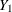,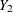, and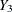(in that order) in a data set, up to eight groups of observations can be formed from the data set. Figure 57.6 displays the eight groups of observations and an unique missing pattern for each group.

Figure 57.6: Missing Data Patterns

 Missing Data Patterns

Group Y1 Y2 Y3
1 X X X
2 X X .
3 X . X
4 X . .
5 . X X
6 . X .
7 . . X
8 . . .

Here, an X means that the variable is observed in the corresponding group and a . means that the variable is missing.

The variable order is used to derive the order of the groups from the data set, and thus determines the order of missing values in the data to be imputed. If you specify a different order of variables in the VAR statement, then the results are different even if the other specifications remain the same.

A data set with variables,, …,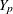(in that order) is said to have a monotone missing pattern when the event that a variable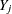is missing for a particular individual implies that all subsequent variables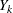,, are missing for that individual. Alternatively, when a variableis observed for a particular individual, it is assumed that all previous variables,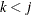, are also observed for that individual.

For example, Figure 57.7 displays a data set of three variables with a monotone missing pattern.

Figure 57.7: Monotone Missing Patterns

 Monotone Missing Data Patterns

Group Y1 Y2 Y3
1 X X X
2 X X .
3 X . .

Figure 57.8 displays a data set of three variables with a non-monotone missing pattern.

Figure 57.8: Non-monotone Missing Patterns

 Non-monotone Missing Data Patterns

Group Y1 Y2 Y3
1 X X X
2 X . X
3 . X .
4 . . X

A data set with an arbitrary missing pattern is a data set with either a monotone missing pattern or a non-monotone missing pattern.